Next: Continuity equation for the Up: Continuity equation in the Previous: Time evolution of a

## Time-dependent density functional theory

In the framework of the time-dependent Hartree-Fock (TDHF) approximation or time-dependent density functional theory (TDDFT), the so-called memory effects are often neglected and it is assumed that the potential at time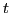is just the static potential evaluated at the instantaneous density [G. F. Giuliani and G. Vignale,The Quantum Theory Of The Electron Liquid, (Cambridge University Press, Cambridge, 2005)()]. For these two time-dependent approaches, the starting point is the equation of motion for the one-body density matrix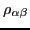[14,13],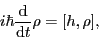(9)

where the mean-field Hamiltonian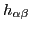is defined as the derivative of the total energy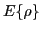with respect to the density matrix,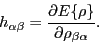(10)

In the present study we are concerned with the Kohn-Sham approach , whereby the total energy is the sum of the kinetic and potential-energy terms,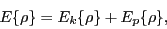(11)

where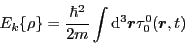(12)

and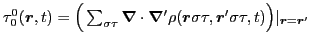is the scalar-isoscalar kinetic density, see, e.g., Ref.  for definitions. The nonlocal density, can be defined in terms of either the fixed-basis orbitals,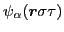,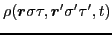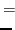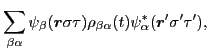(13)

or instantaneous Kohn-Sham orbitals,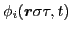,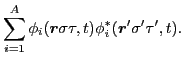(14)

The mean-field Hamiltonian is the sum of kinetic and potential-energy terms,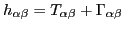, where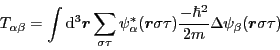(15)

and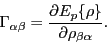(16)

Let us now assume that the potential energy is invariant with respect to a unitary transformation of the density matrix [14,13],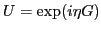, that is, for all parameters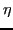we have,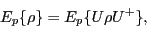(17)

where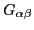is the hermitian matrix of a one-body symmetry generator. Then, the first-order expansion in,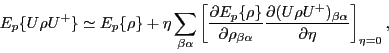(18)

gives a condition for the energy to be invariant with respect to this unitary transformation, that is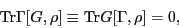(19)

which allows us to derive the equation of motion for the average value of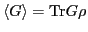. Indeed, from the TDDFT equation (9) we then have: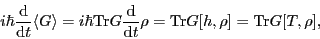(20)

that is, the time evolution of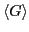is governed solely by the kinetic term of the mean-field Hamiltonian.

SubsectionsNext: Continuity equation for the Up: Continuity equation in the Previous: Time evolution of a
Jacek Dobaczewski 2011-11-11